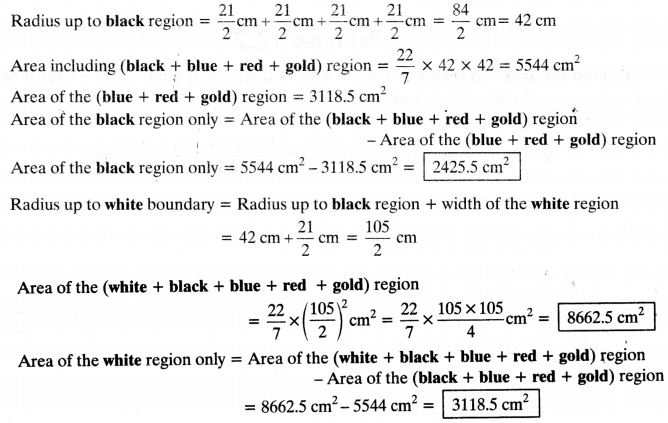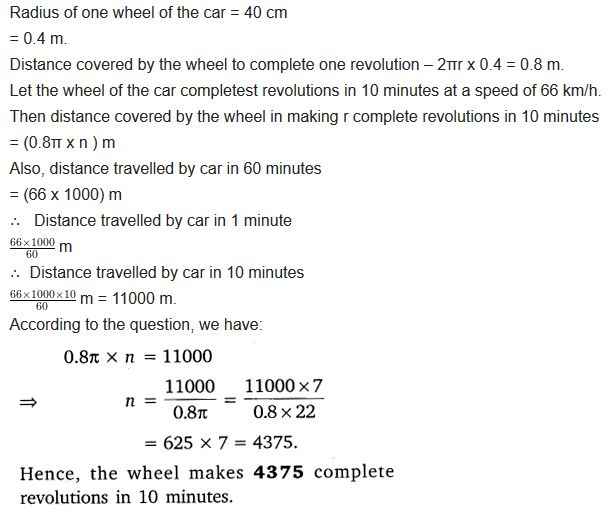ncert textbook

# NCERT Solutions for Class 10 Maths Chapter 12 Areas Related to Circles Ex 12.1

Get Free NCERT Solutions for Class 10 Maths Chapter 12 Ex 12.1 PDF. Areas Related to Circles Class 10 Maths NCERT Solutions are extremely helpful while doing your homework. Exercise 12.1 Class 10 Maths NCERT Solutions were prepared by Experienced ncert-books.in Teachers. Detailed answers of all the questions in Chapter 12 Maths Class 10 Areas Related to Circles Exercise 12.1 provided in NCERT TextBook.Question 1.
The radii of the two circles are 19 cm and 9 cm respectively. Find the radius of the circle which has a circumference equal to the sum of the circumferences of the two circles.
Solution:Question 2.
The radii of two circles are 8 cm and 6 cm respectively. Find the radius of the circle having area equal to the sum of the areas of the two circles.
Solution:Question 3.
The given figure depicts an archery target marked with its five scoring regions from the center outwards as Gold, Red, Blue, Black, and White.The diameter of the region representing Gold score is 21 cm and each of the other bands is 10.5 cm wide. Find the area of each of the five scoring regions.
Solution:Question 4.
The wheels of a car are of diameter 80 cm each. How many complete revolutions does each wheel make in 10 minutes when the car is travelling at a speed of 66 km per hour?
Solution:Question 5.
Tick the correct answer in the following and justify your choice: If the perimeter and the area of a circle are numerically equal, then the radius of the circle is
(a) 2 units
(b) n units
(c) 4 units
(d) 7 units
Solution: